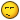BrainDen.com - Brain Teasers
• 0

## Question

Thought this was cool, but i didnt know where to post it, so here it is!

[sqrt(x)]=the square root of x

Given:

[sqrt(a)(b)]=[sqrt(a)][sqrt(b)]

[sqrt(-1)]=i

1+1

=1+[sqrt(1)]

=1+[sqrt(-1)(-1)]

=1+[sqrt(-1)][sqrt(-1)]

=1+(i)(i)

=1+i2

=1+(-1)

=0

yeah, i know there is a small flaw, but i still think its pretty cool!

Edited by MissKitten

## Recommended Posts

• 0It is sooooo true!!!##### Share on other sites
• 0

yeah, i know. as soon as i can remember how to make 2x2=5, ill post that too.

##### Share on other sites
• 0[sqrt(a)(b)]=[sqrt(a)][sqrt(b)]

It is pretty cool. I wonder what percentage of high school students know that the rule that sqrt((a)(= sqrt(a)sqrt(b) only applies when a and b are non-negative real numbers (that's the hidden mistake for those who weren't sure). I myself hadn't considered it. I only realized it when following the work to show that 1+1=0.

##### Share on other sites
• 0

Party pooper.jk!

## Join the conversation

You can post now and register later. If you have an account, sign in now to post with your account.×   Pasted as rich text.   Paste as plain text instead

Only 75 emoji are allowed.

×   Your previous content has been restored.   Clear editor

×   You cannot paste images directly. Upload or insert images from URL.

×